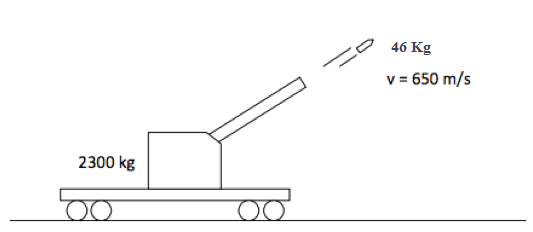Classical Mechanics

# Momentum 2D - Problem Solving

Water with density $1.00 \times 10^3 \text{ kg/m}^3$ streams from a hose $3.00\text{ cm}$ in diameter, in a horizontal jet at a constant rate of $19.0 \text{ m/s}.$ It horizontally strikes a nearby vertical sponge-like water-absorbing wall, thereupon transferring all its kinetic energy to the wall so there is no splashing. What is the magnitude of the force exerted by the water on the wall?

A toy that is initially at rest consists of three parts that are held together by spring-loaded clips. Suddenly the toy is broken into pieces because of loosened clips, and two of the pieces which have equal masses of $26.0 \text{ g}$ travel with velocities of $4.0 \text{ cm/s},$ one to the south and the other to the west. If the third piece travels with a velocity of $2.0 \sqrt{2} \text{ cm/s}$ due northeast, what is the mass of the third piece?

A projectile proton with a speed of $240 \text{ m/s}$ collides elastically with a target proton initially at rest. The two protons then move along perpendicular paths, with the projectile path deviating by $60 ^\circ$ from its original direction. After the collision, what is the approximate speed of the target proton?

A $1560 \text{ kg}$ car heading west at $12 \text{ m/s}$ turns right at a corner and travels north at $9 \text{ m/s}.$ Find the magnitude of the change in the car's momentum.A $2300 \text{ kg}$ cannon mounted on a railway car fires a $46 \text{ kg}$ shell at a velocity of $650 \text{ m/s}$ at an angle of $60^{\circ}$ above the horizontal. What is the magnitude of recoil velocity of the cannon?

Details: ignore the effects of friction.

×